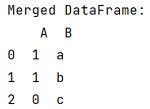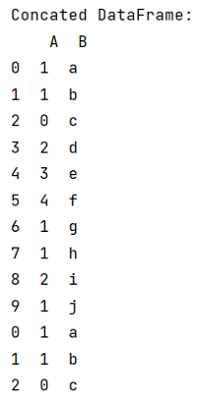# Difference between merge() and concat() in pandas

Learn, what are the difference between merge() and concat() in pandas? By Pranit Sharma Last updated : September 23, 2023

Pandas is a special tool that allows us to perform complex manipulations of data effectively and efficiently. Inside pandas, we mainly deal with a dataset in the form of DataFrame. DataFrames are 2-dimensional data structures in pandas. DataFrames consist of rows, columns, and the data.

Pandas provide numerous ways to combine two Series or DataFames to perform effective and efficient data analytics. Sometimes our required data is not present in a single DataFrame and in that case we need to combine two or more DataFrames.

## Difference between merge() and concat()

Pandas merge() and pandas concat() are both the methods of combining or joining two DataFrames but the key difference between the merge() and join() method is that the merge() method works based on common value and combines the DataFrame while on the other hand concat() is also used to combine DataFrames but it is a method which appends or inserts one (or more) DataFrame below the other.

Note

To work with pandas, we need to import pandas package first, below is the syntax:

```import pandas as pd
```

Let us understand with the help of an example,

## Python program for understand the difference between merge() and concat() in pandas

```# Importing pandas package
import pandas as pd

# Creating dictionary
d1 = {
'A':[1,1,0,2,3,4,1,1,2,1],
'B':['a','b','c','d','e','f','g','h','i','j']
}

d2 = {
'A':[1,1,0],
'B':['a','b','c']
}

# Creating  dataframe
df1 = pd.DataFrame(d1)
df2 = pd.DataFrame(d2)

# Display DataFrames
print("Created DataFrame 1:\n",df1,"\n")
print("Created DataFrame 2:\n",df2,"\n")

# Using merge
result = pd.merge(df1, df2)

# Display result
print("Merged DataFrame:\n",result)

# Using concat()
result = pd.concat([df1, df2])

# Display result
print("Concated DataFrame:\n",result)
```

### Output

After applying merge() method:After applying concat() method: